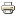# From Observable Behaviors to Structures of Interaction in Binary Games of Strategic ComplementsArticle
Author/s:
Tomás Rodríguez Barraquer
Entropy
Issue number:
Volume 15, Issue 11
Publisher:
MDPI
Year:
2013
Journal pages:
4648-4667
Consider a setting in which agents can take one of two ordered actions and in which the incentive to take the high action increases in the number of other agents taking it. Furthermore, assume that we do not know anything else about the game being played. What can we say about the details of the interaction between actions and incentives when we observe a set or a subset of all possible equilibria? In this paper, we study this question by exploring three nested classes of games: (a) binary games of strategic complements; (b) games in (a) that admit a network representation; and (c) games in (b) in which the network is complete. Our main results are the following: It has long been established in the literature that the set of pure strategy Nash equilibria of any binary game of strategic complements among a set, N, of agents can be seen as a lattice on the set of all subsets of N under the partial order defined by the set inclusion relation (C). If the game happens to be strict in the sense that agents are never indifferent among outcomes (games in (a)), then the resulting lattice of equilibria satisfies a straightforward sparseness condition. (1) We show that, in fact, for each such lattice, L, there is a game in (a), such that its set of equilibria is L (we say that such a game expresses L); (2) We show that there exists a game in (b), whose set of equilibria contains a given collection, C, of subsets of N, if and only C satisfies the sparseness condition, and the smallest game in (a) expressing C is trade robust; (3) We show that there exists a game on the complete graph (games in (c)), whose set of equilibria coincides with some collection, C, if and only if C is a chain satisfying the sparseness condition.
Developed by Paolo Gittoi